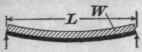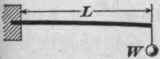## Then, 236,210 Ft

lb. - 112,250 ft.-lb. = 123,960 ft.-lb., the maximum bending moment. The bending moment in inch-pounds is 123,960 ft.-lb. X 12 = 1,487,520 in.-lb

If W= the load and L = the span, the maximum bending moment in a simple beam uniformly loaded is (W / 2)x(L/2)

- (W/2)x(L/4)= WL/8.(L/4 is the distance from the center of beam to center of gravity of each half of the uniform load.)

The maximum bending moment in a beam with a load con eentrated at the center is WL/4. Thus, a beam uniformly loaded will carry safely twice as much as if the. load were concentrated at the center.

While, by the preceding principles, the bending moment in any beam supported at one or two supports may be deter mined, it is more convenient to use concise formulas. The following table gives formulas for the maximum bending moment, maximum safe loads, and greatest deflections (or sag), for beams loaded and supported in different ways:

## Table XXVI

 Method of Loading. MaximumBendingMoment.if. Maximum Load. W. Deflection. D. Length in Load in Feet. Pounds. Ft.-Lb. In.-Lb. Lb. In.WL/8 3WL/2 2QS/3L 5Wl3/384EIWL/6 2WL QS/2L Wl3/60EIWL/2 6WL QS/6L Wl3/8EIWL 12WL QS/12L Wl3/3EIWL 4 3WL QS/3L Wl3/48EIWA/2 6WA QS/6A (Wa/48EI)X (3l2-4a2) Between Supports. WA/2 6WA Q S/6A (Wa/16EI) X(I -2 a)2

L = length in feet; I = length in inches; W= total load in pounds; E = modulus of elasticity; I = moment of inertia; Q = section modulus; S = safe stress on the extreme fibers of the beam section (= modulus of rupture / factor of safety). In figuring deflections, all lengthsmust be expressed in inches; and small letters I, a, and b are used as reminders.